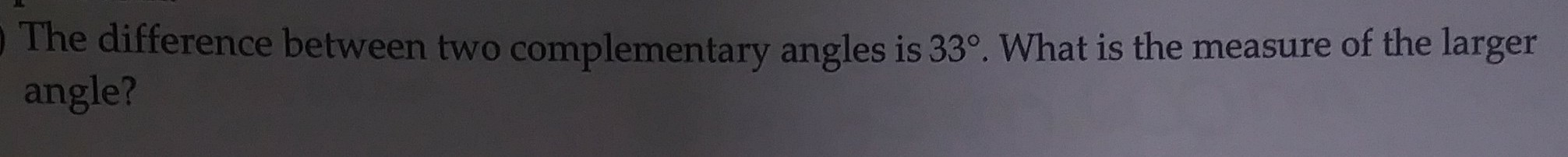# The difference between two complementary angles is 33°. What is the measure of the largerangle?

Question
4 viewshelp_outlineImage TranscriptioncloseThe difference between two complementary angles is 33°. What is the measure of the larger angle? fullscreen
check_circle

Step 1

Let A and B be the  two complementary angles where A is larger than B.

Since A and B are complementary angles , so their sum is 90 degrees.

Step 2

Also, according to given condition , we have

Step 3

By adding the two equations ,...

### Want to see the full answer?

See Solution

#### Want to see this answer and more?

Solutions are written by subject experts who are available 24/7. Questions are typically answered within 1 hour.*

See Solution
*Response times may vary by subject and question.
Tagged in

### Other